Nothing special here. It’s just a blog post for summarising my algorithm learning course. Probably this was taught in the University but I don’t remember anything, I have no idea about its definition and applications until I take this course. Part 1 here Binary Heap & Heapsort Summary - Part 1 - Binary Heap

# The Idea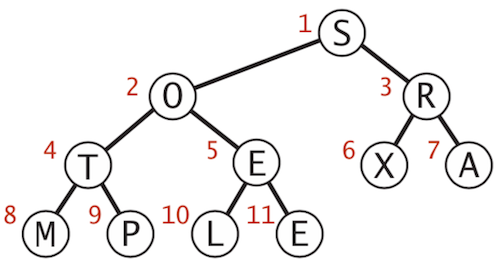• Create max-heap with all N keys.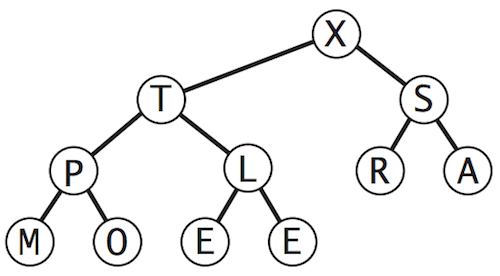• Repeatedly remove the maximum key (in place) to create a sorted array.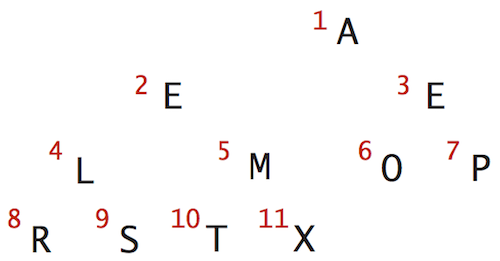# First step: Heap construction

Build heap using bottom-up method. Start with the lowest nodes and go up each level, use sink operation to correct the heap.

• Starting point (arbitrary order)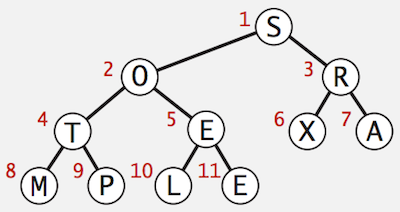• All the nodes in the lowest level are 1-node binary heap. In this case E, L, P and M are already in sorted order (1-node binary heaps).
• Start with the nodes in the upper level, X, A, E and T in this case
• X and A are already 1-node binary heaps
• Apply sink(5) operation on E to make it a sorted binary heap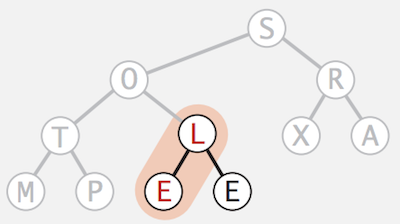• Apply sink(4) operation on T, nothing to do here because it’s already a sorted binary heap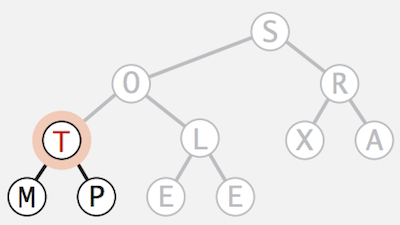• Continue with the nodes in higher level, X and O in this case
• Apply sink(3) operation on R to make it a sorted binary heap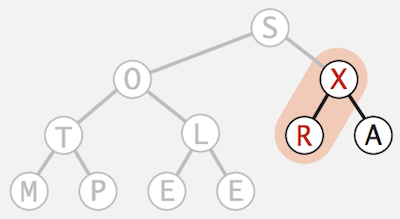• Apply sink(2) operation on O to make it a sorted binary heap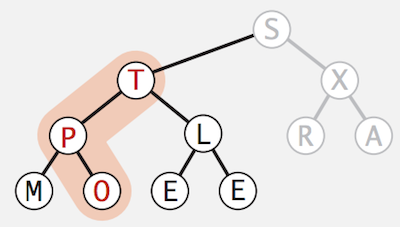• Continue with the node in the highest level, S in this case
• Apply sink(1) operation on S to make it a sorted binary heap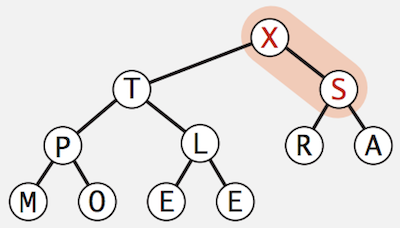• We finally transform an arbitrary array into a heap-ordered array
for (int k = N/2; k >= 1; k--)
sink(a, k, N);


# Second step: Sortdown

In order to transform a heap-ordered array into a sorted array, we will repeatedly remove the largest item in the heap, one at a time. Refer to part 1 for the idea on how to remove the maximum item in a heap. The only difference is that after exchanging the max with the last item, we will keep it in the array instead of completely removing it out.

• Starting point (a heap-ordered array)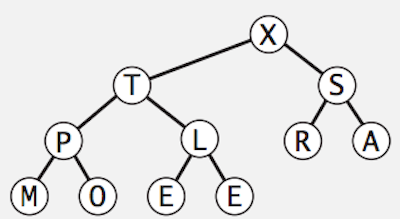• Repeatedly remove the largest item, once at a time, keep it at the end of the array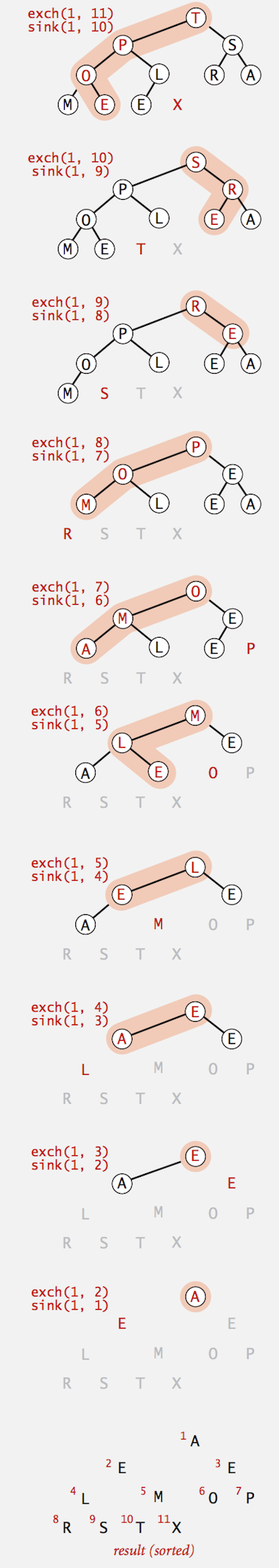while (N > 1) {
swap(a, 1, N--);
sink(a, 1, N);
}


# Java Implementation

public class Heap {
public static void sort(Comparable[] a) {
int N = a.length;
// build the heap
for (int k = N/2; k >= 1; k--)
sink(a, k, N);

// convert heap to sorted array
while (N > 1)
{
exch(a, 1, N);
sink(a, 1, --N);
}
}

private static void sink(Comparable[] a, int k, int N) {
// implemented in part 1
}

private static boolean less(Comparable[] a, int i, int j) { /* compare */ }

private static void swap(Comparable[] a, int i, int j) { /* swap */ }
}


# Heapsort Characteristics

• Heapsort is an In-place sorting algorithm with N logN worst-case
• Compare to the other sorting algorithm
• Mergesort: not in-place, linear extra space required.
• Quicksort: in-place, but quadratic time in worst case.
• Heapsort is optimal for both time and space, but:
• Inner loop longer than Quicksort’s
• Makes poor use of cache memory